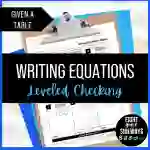Math > Algebra | Grade 9, 10, 11, 12 | Worksheets

# Writing Linear Equations (from Tables) - Leveled Checking WorksheetAttributes
Subject

Algebra

Types

Worksheets

File

PDF

Editable
No
RatingThis is a Writing Linear Equations (from Tables) - Leveled Checking Worksheet.

There are 4 sets with 3 problems each.

For Sets A and B, students will complete each problem and match it to an answer in the “Answer Bank.” Then they will write the corresponding letter in the appropriate spot.

For Set C, a proposed answer is given. They will determine if the proposed Set C: answer is correct or incorrect and show all work that led them to their decision.

For Set D, they will complete each problem and show all their work.

4-page PDF

## Resource Tags

algebra writing equations linear quations tables graphing

## 0 Reviews

### you may also like...

Check out these other great products